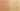StringPlusStringifiesExpression

Misconception:

A plus with a String as one operand and an expression as another operand concatenates the string and a string representation of the expression (e.g., "value:" + (1 - 3) evaluates to something like "value: (1 - 3)".Incorrect

String concatenation stringifies non-String operand expressions

Correct

String concatenation evaluates non-String operand expressions and casts value to String

CorrectionHere is what's right.

The plus operator concatenates two strings. First, both operands are evaluated. If the type of one of the operands is not String, that value is converted to a string, after being evaluated, and before being concatenated.

ValueHow can you build on this misconception?

This misconception isn’t as far-fetched as it might first look.

Relationship to S-Expressions, Quote, and External Representation

Representing code as data is an idea that goes back to early languages like Lisp, and later Scheme or Racket, which use S-expressions to represent code as well as data, and allow programs to represent and modify code.

To represent any S-expression as data, one can simply prefix it with a quote. Thus, (write '(+ 1 2)) will not evaluate the (+ 1 2) but will print (+ 1 2), while (write (+ 1 2)) will evaluate the (+ 1 2) and will print 3.

Moreover, Lisp uses the concept of “external representation”, which essentially is a representation of an expression as a sequence of characters. So "(+ 1 2)" is the external representation of the expression (+ 1 2).

The idea of quoting some code is closely related to the idea of stringifying the argument of a string concatenation.

Relationship to Unevaluated Operands

The idea that the String concatenation operator does not evaluate one of its operands, but instead somehow works with the unevaluated expression is related to the idea of “unevaluated expressions” or “unevaluated operands”. Some programming languages provide so-called “unevaluated expressions”, and operators that do not actually evaluate their operands. For example, the C++ sizeof operator does not evaluate its operand but simply determines its static type.

struct Empty {};
Empty empty;
std::size_t size = sizeof empty; // size of an Empty
size = sizeof int;           // size of an array of 10 ints

Note that while this does not evaluate the operand, it also does not represent the operand as some runtime structure. Thus, it’s still considerably different from the idea of a stringified expression.

Relationship to Call-by-Name

The idea also is somewhat related to call-by-name, where the argument to a function call is passed as an unevaluated expression and then evaluated in the called function each time it is used. Here is an example in a made-up C-like language:

int i = 0;
char a = { 19, 42 };

f(a[i]);     // call-by-name -- pass unevaluated "a[i]" to f

int f(int arg) {
int k = arg; // evaluates a[i], currently a = 19
i = 1;       // changes i
k = arg;     // evaluates a[i], currently a = 42
}

Note that while this does not evaluate the operand before the call, it also does not represent the operand as some runtime structure, and it simply defers evaluation to the execution of the callee’s body. Thus, it’s still considerably different from the idea of a stringified expression.

Java